## a fly undergoes a displacement of – 5.80 while accelerating at -1.33 m/s^2 for 4.22 s. what was the initial velocity of the fly?

Question

a fly undergoes a displacement of – 5.80 while accelerating at -1.33 m/s^2 for 4.22 s. what was the initial velocity of the fly?

in progress 0
6 months 2021-08-18T16:44:08+00:00 1 Answers 0 views 0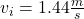Explanation:

The computation of the initial velocity of the fly is shown below:-

But before that we need to do the following calculations

For 4.22 seconds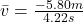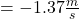For uniform acceleration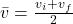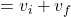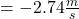With initial and final velocities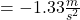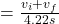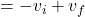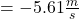So, the initial velocity isWe simply applied the above steps to reach at the final solution i.e initial velocity